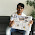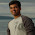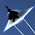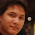## January 28, 2010

### Parsing Excel Sheet made easy with Annotations and POI

HSSF - Horrible Spreadsheet Format – not anymore. With few annotations, excel parsing can be done in one line.

We had a requirement in our current project to parse multiple excel sheets and store the information to database. I hope most of the projects involving excel sheet parsing would be doing the same. We built a extensible framework to parse multiple sheets and populate JAVA objects with annotations.

We will discuss the steps to use annotations to parse excel sheet and populate Java objects.

Consider we have an excel sheet with student information.
Parsing Logic:
While parsing this excel sheet, we need to populate one “Section” object and multiple “Student” objects related to a Section. You can see that Student information is available in multiple rows whereas the Section details (Year, Section) is available in column B.

We will have to annotate the above information to the domain class, that can be interpretted by the sheet parser.

1) Annotate Domain Class:
First we will see the steps to annotate Section object:

``````@ExcelObject(parseType = ParseType.COLUMN, start = 2, end = 2)
public class Section {
@ExcelField(position = 2)
private String year;

@ExcelField(position = 3)
private String section;

@MappedExcelObject
private List <Student> students;
}``````
You can find three different annotation in this class.
i)ExcelObject: This annotation tells the parser about the parse type (Row or Column), number of objects to create (start, end). Based on the above annotation, Section value should be parsed Columnwise and information can be found in Column 2 (“B”) of the Excelsheet.

ii)ExcelField: This annotation tells the parser to fetch “year” information from Row 2 and “section” information from Row 3.

iii)MappedExcelObject: Apart from Simple datatypes like “Double”,”String”, we might also try to populate complex java objects while parsing. In this case, each section has a list of student information to be parsed from excel sheet. This annotation will help the parser in identifying such fields.

Next step is to annotate Student class:

``````@ExcelObject(parseType = ParseType.ROW, start = 6, end = 8)
public class Student {
@ExcelField(position = 2)
private Long roleNumber;

@ExcelField(position = 3)
private String name;

@ExcelField(position = 4)
private Date dateOfBirth;

@ExcelField(position = 5)
private String fatherName;

@ExcelField(position = 6)
private String motherName;

@ExcelField(position = 7)
private String address;

@ExcelField(position = 8)
private Double totalScore;
}``````
i) ExcelObject: As shown above, this annotation tells parser to parse Rows 6 to 8 (create 3 student objects). NOTE: Optional field “zeroIfNull” , if set to true, will populate Zero to all number fields (Double,Long,Integer) by default if the data is not available in DB.

ii) ExcelField: Student class has 7 values to be parsed and stored in the database. This is denoted in the domain class as annotation.

iii) MappedExcelObject: Student class does not have any complex object, hence this annoation is not used in this domain class.

Once the annotation is done, you have just invoke the parser with the Sheet and the Root class you want to populate.

``````//Get the sheet using POI API.
String sheetName = "Sheet1";
SheetParser parser = new SheetParser();
InputStream inputStream = getClass().getClassLoader().getResourceAsStream("Student Profile.xls");
Sheet sheet = new HSSFWorkbook(inputStream).getSheet(sheetName);

//Invoke the Sheet parser.
List entityList = parser.createEntity(sheet, sheetName, Section.class);
``````

Thats all it requires. Parser would populate all the fields based on the annotation for you.

You can find the source code in Github

#### 8 comments:

1.This comment has been removed by the author.

2.We have the same scenario in our project,But slight changes in entry in excel.

i.e.
In the above example;If Section and Student data entry repeats in the sheet again, How do we create the Map for It Example.
@ExcelObject(parseType = ParseType.ROW, start = 1, end = 2000)
class RepeatedSectionStudent{
@MappedExcelObject
Section section;
@MappedExcelObject
Student student;
}

But we are getting the same data in next List element also.
Example list.get(0) RepeatedSectionStudent bean value & list.get(1) bean value are same.

Please guide , If I am missing anything.

Thanks
Radha

3.Hi Radha,

Currently the framework asks you for exact positions. Since the Section object has exact positions, you would get the same value in the list.

I was planning to enhance this but did not find time.

4.That was cool. Can you share the source code of your framework?
Thanks

5.Hi Faissal,

You can find the source code @ https://github.com/nvenky/excel-parser

1.Thanks :). I'm trying to parse a "scrambled" sheet not like the Student and Section example where you can see the sheet as a regular POJO. Any pointers?!
Thanks.

6.Hi,

Is there a dynamic way of identifying the total number of rows in a given excel file?

7.Hi Timothy,

I don't remember any quick way to find the total number of rows. I think we used some logic like, if couple of rows are empty then stop processing.